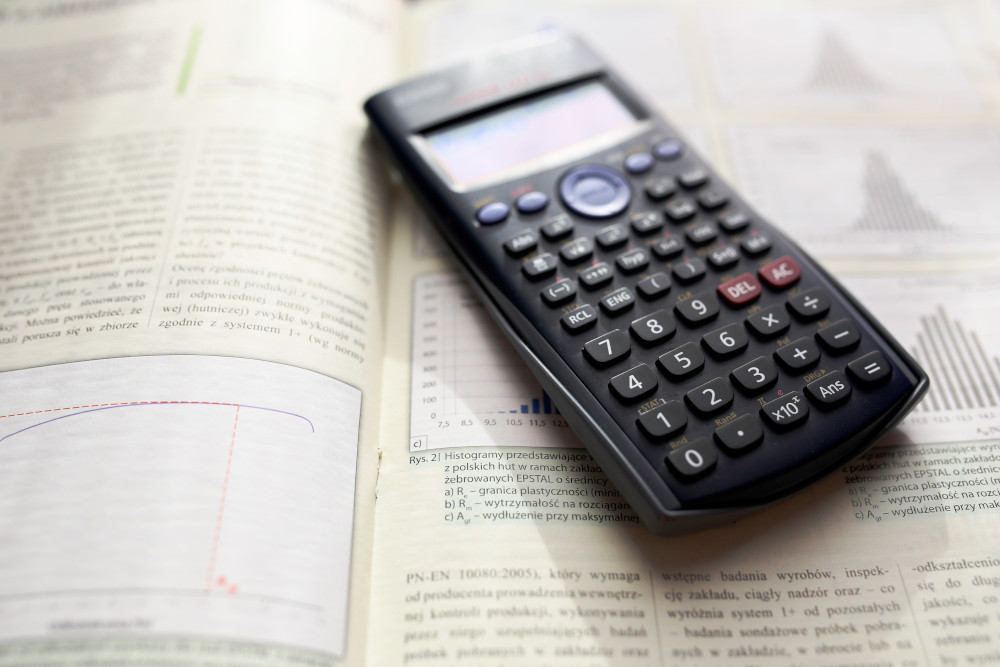# Basic Statistical Concepts for Data ScienceAs a data scientist, it is important to have a deep understanding of statistics. Here, I introduce basic statistical concepts and quantities.

## Types of measurements and variables

Important statistical concepts include the following:

• Types of measurement scales
• Nomenclature for variables: dependent vs independent variables

## Statistical quantities

You should definitely know about the following, frequently used statistical quantities:

• Centrality measures: mean and median, mode
• Measure of dispersion: standard deviation, variance, covariance, interquartile-range
• Interval estimates: confidence intervals

## Probability distributions

Commonly occuring probability distributions are:

• Uniform distribution: all values are equally likely
• Normal distribution: a bell-shaped curve, typical for many population characteristics (e.g. IQs, heights)
• Poisson distribution: an integer distribution that is ideal for count data
• Exponential distribution: a heavy-tailed distribution

## Posts on basic statistics

You can find eplanations of basic statistical concepts and their use in R in the following posts.

### Statistical Nomenclature for Variables

0
Variables can be differentiated by two characteristics. The first characteristic is the scale of the variable (i.e. the values that the variable can assume). The second is the role that the variable fulfills in a statistical model. Measurements scales of variables Variables can be on the following scales: Quantitative variables: Variables indicating numeric values for which pairwise differences are meaningful. Categorical variables: Variables representing a discrete set of groups.

### Using probability distributions in R: dnorm, pnorm, qnorm, and rnorm

1

R is a great tool for working with distributions. However, one has to know which specific function is the right wrong. Here, I’ll discuss which functions are available for dealing with the normal distribution: dnorm, pnorm, qnorm, and rnorm.

### Mean vs Median: When to Use Which Measure?

1

Two of the most commonly used statistical measures are the mean and the median. Both measures indicate the central value of a distribution, that is, the value at which one would expect the majority of data points to lie. In many applications, however, it is useful to think about which of the two measures is more appropriate given the data at hand. In this post, we’ll investigate the differences between both quantities and give recommendations when one should be preferred over the other.